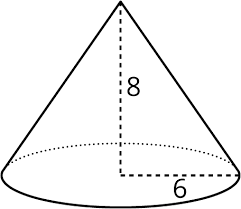# C Program To Find Volume Of Cone | C Programs

C Program to find the volume of a cone – In this distinct article, we will brief in on all the ways to find the volume of a cone using C programming.

We have added suitable examples and sample programs to the blog post as well. The compiler has been added for an easy understanding of the whole setup.

The methods that have been used to calculate the volume of a cone in C programming are as follows:

• Using Standard Method
• Using Functions
• Using Pointers
• Using Macros

As we all know, a cone is a three-dimensional figure. It is an important figure in use in our daily lives. A cone has a circular base and tapers off into a single point.

From a two-dimensional perspective, it looks like a triangle. A cone looks like this:As you can see, this is a cone with a radius of 6 units and a height of 8 units.

In order to calculate the volume of a cone, we need to use this formula:

Volume = 1/3 * Pi * r^2 * h

Hence, the volume is this cone is as follows:

Volume = 1/3 * 22/7 * 6^2 * 8 = 301.59 sq. units.

Thus, the ways to calculate the volume of a cone in C programming are as follows:

## Using Standard Method

1)We are using the standard formula for calculating the volume of the cone. Here, we need radius and height of the cone.

2)The values of radius and height will store into the variables “radius” and “height”, these values substitute into the formula we will get the volume of a cone, that value will store into variable “volume”.

output:

### Using Functions

1)Here volume(r,h)is calling function,and volume(float r,float h) is called function.

2)We are calling the function using volume(r,h).

3)The called function volume(float r,float h) calculate the volume and returns the value, that value will store into variable “v”.

output:

#### Using Pointers

1)Here *r,*h,*v are the pointer variables

2)We are calling the function using volume(&r,&h,&v) by passing addresses as arguments.

3)The called function volume(float *r,float *h,float *v) will calculate the volume ,that volume value will store into  pointer variable v.

output:

##### Using Macros

1)volume(r,h) is the symbolic name to the formula  (22*r*r*h)/(3*7)

2)volume(r,h)replaced with that expression given at #define.

output:
x

## C Program To Print Number Of Days In A Month | Java Tutoring

C program to input the month number and print the number of days in that ...